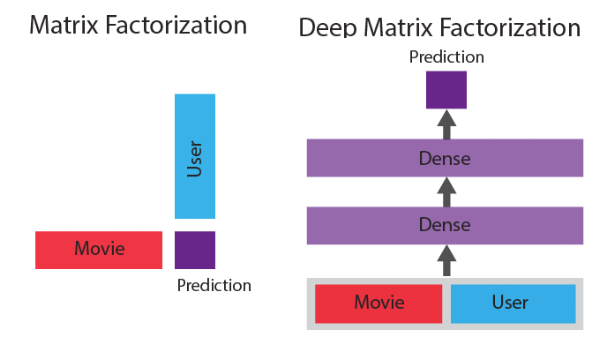## Monday, July 26, 2021

### Data Science Study Notes: Pandas user-defined function(UDF)

3 Methods for Parallelization in Spark
Native Spark: if you’re using Spark data frames and libraries (e.g. MLlib), then your code we’ll be parallelized and distributed natively by Spark.

Thread Pools: The multiprocessing library can be used to run concurrent Python threads, and even perform operations with Spark data frames. Using thread pools this way is dangerous, because all of the threads will execute on the driver node. If possible it’s best to use Spark data frames when working with thread pools, because then the operations will be distributed across the worker nodes in the cluster.

The threading module uses threads, the multiprocessing module uses processes. The difference is that threads run in the same memory space, while processes have separate memory. This makes it a bit harder to share objects between processes with multiprocessing. Since threads use the same memory, precautions have to be taken or two threads will write to the same memory at the same time. This is what the global interpreter lock is for.

Using subprocess,multiprocess or multi threads in the pyspark environment might easily cause some errors: function not exists on the JVM, it might be perfectly fine if you run on process! But not available on the multiple process run parallel.

``````# spark version
from pyspark.ml.regression import RandomForestRegressor

# define a function to train a RF model and return metrics
def mllib_random_forest(trees, boston_train, boston_test):

# train a random forest regressor with the specified number of trees
rf = RandomForestRegressor(numTrees = trees, labelCol="target")
model = rf.fit(boston_train)

# make predictions
boston_pred = model.transform(boston_test)
r = boston_pred.stat.corr("prediction", "target")

# return the number of trees, and the R value
return [trees, r**2]

pool.map(lambda trees: mllib_random_forest(trees, boston_train, boston_test), parameters)
``````
Powerful Pandas UDFs: A new feature in Spark that enables parallelized processing on Pandas data frames within a Spark environment.

Note: Pandas UDF is not the same as Python UDF. Pandas UDF has much better performance.
A pandas user-defined function (UDF)—also known as vectorized UDF—is a user-defined function that uses Apache Arrow to transfer data and pandas to work with the data. pandas UDFs allow vectorized operations that can increase performance up to 100x compared to row-at-a-time Python UDFs.

Two types of Pandas UDF: Grouped Map Pandas UDF vs Scalar Pandas UDFs

To define a scalar Pandas UDF, simply use @pandas_udf to annotate a Python function that takes in pandas.Series as arguments and returns another pandas.Series of the same size. Below we illustrate using two examples: Plus One and Cumulative Probability.
``````
#Using row-at-a-time UDFs, very slow:

from pyspark.sql.functions import udf

# Use udf to define a row-at-a-time udf
@udf('double')
# Input/output are both a single double value
def plus_one(v):
return v + 1

df.withColumn('v2', plus_one(df.v))

#Using Pandas UDFs, much faster:

from pyspark.sql.functions import pandas_udf, PandasUDFType

# Use pandas_udf to define a Pandas UDF
@pandas_udf('double', PandasUDFType.SCALAR)
# Input/output are both a pandas.Series of doubles

def pandas_plus_one(v):
return v + 1

df.withColumn('v2', pandas_plus_one(df.v))
``````
The examples above define a row-at-a-time UDF “plus_one” and a scalar Pandas UDF “pandas_plus_one” that performs the same “plus one” computation. The UDF definitions are the same except the function decorators: “udf” vs “pandas_udf”.

Grouped Map Pandas UDFs
Python users are fairly familiar with the split-apply-combine pattern in data analysis. Grouped map Pandas UDFs are designed for this scenario, and they operate on all the data for some group, e.g., “for each date, apply this operation”.

Grouped map Pandas UDFs first splits a Spark DataFrame into groups based on the conditions specified in the groupby operator, applies a user-defined function (pandas.DataFrame -> pandas.DataFrame) to each group, combines and returns the results as a new Spark DataFrame.

Subtract Mean: This example shows a simple use of grouped map Pandas UDFs: subtracting mean from each value in the group.

``````
@pandas_udf(df.schema, PandasUDFType.GROUPED_MAP)
# Input/output are both a pandas.DataFrame
def subtract_mean(pdf):
return pdf.assign(v=pdf.v - pdf.v.mean())

df.groupby('id').apply(subtract_mean)

In this example, we subtract mean of v from each value of v for each group.
The grouping semantics is defined by the “groupby” function,
i.e, each input pandas.DataFrame to the user-defined function has the same “id” value.
The input and output schema of this user-defined function are the same,
so we pass “df.schema” to the decorator pandas_udf for specifying the schema.

Grouped map Pandas UDFs can also be called as standalone Python functions on the driver.
This is very useful for debugging, for example:

sample = df.filter(id == 1).toPandas()
# Run as a standalone function on a pandas.DataFrame and verify result
subtract_mean.func(sample)

# Now run with Spark
df.groupby('id').apply(substract_mean)
``````
In the example above, we first convert a small subset of Spark DataFrame to a pandas.DataFrame, and then run subtract_mean as a standalone Python function on it. After verifying the function logics, we can call the UDF with Spark over the entire dataset.

Ordinary Least Squares Linear Regression
The last example shows how to run OLS linear regression for each group using statsmodels. For each group, we calculate beta b = (b1, b2) for X = (x1, x2) according to statistical model Y = bX + c.

``````
import statsmodels.api as sm
# df has four columns: id, y, x1, x2

group_column = 'id'
y_column = 'y'
x_columns = ['x1', 'x2']
schema = df.select(group_column, *x_columns).schema

@pandas_udf(schema, PandasUDFType.GROUPED_MAP)
# Input/output are both a pandas.DataFrame
def ols(pdf):
group_key = pdf[group_column].iloc
y = pdf[y_column]
X = pdf[x_columns]
model = sm.OLS(y, X).fit()

return pd.DataFrame([[group_key] + [model.params[i] \
for i in   x_columns]], columns=[group_column] + x_columns)

beta = df.groupby(group_column).apply(ols)
``````
This example demonstrates that grouped map Pandas UDFs can be used with any arbitrary python function: pandas.DataFrame -> pandas.DataFrame. The returned pandas.DataFrame can have different number rows and columns as the input.